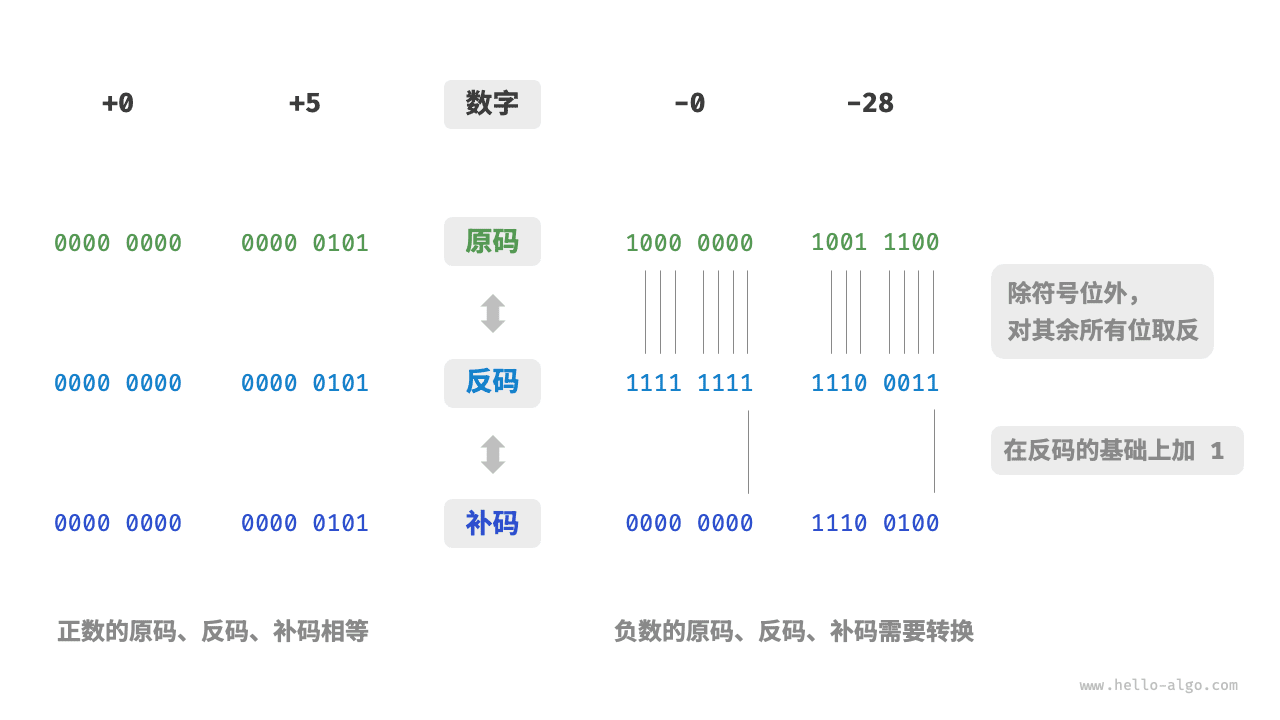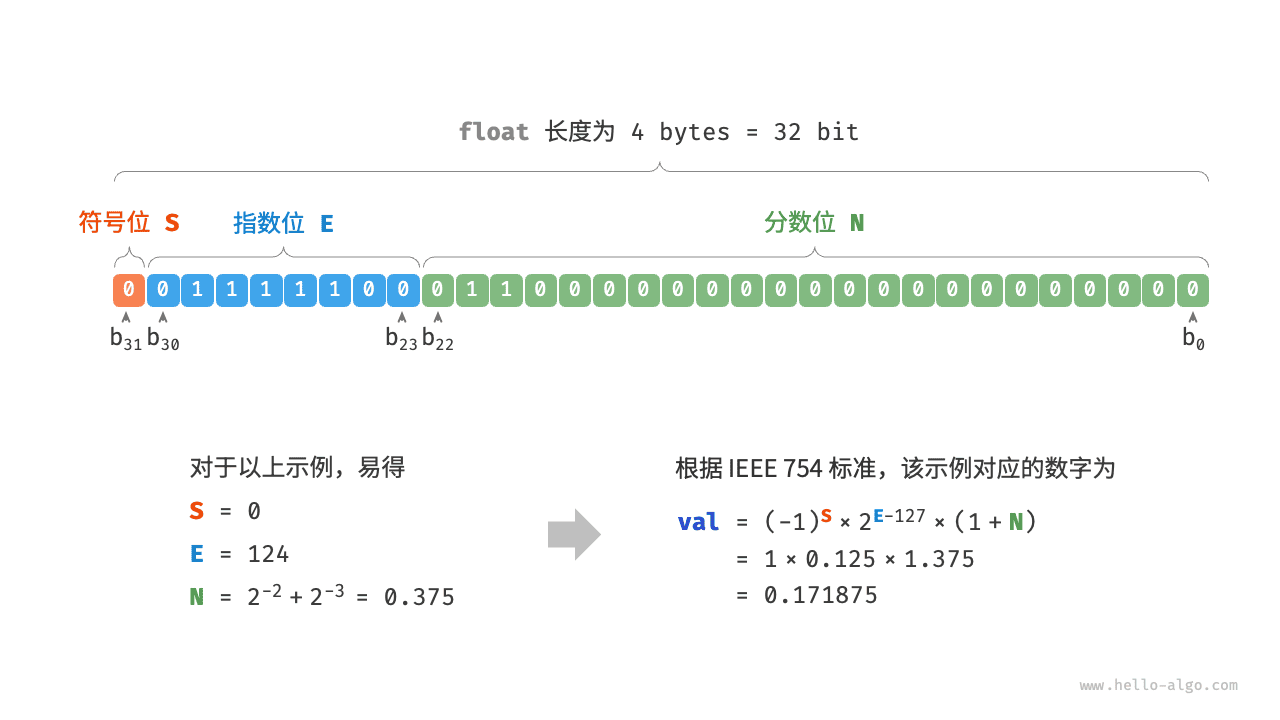# 3.3   数字编码 *¶

Note

## 3.3.1   整数编码¶

• 原码：我们将数字的二进制表示的最高位视为符号位，其中 $$0$$ 表示正数，$$1$$ 表示负数，其余位表示数字的值。
• 反码：正数的反码与其原码相同，负数的反码是对其原码除符号位外的所有位取反。
• 补码：正数的补码与其原码相同，负数的补码是在其反码的基础上加 $$1$$「原码 sign–magnitude」虽然最直观，但存在一些局限性。一方面，负数的原码不能直接用于运算。例如在原码下计算 $$1 + (-2)$$ ，得到的结果是 $$-3$$ ，这显然是不对的。

\begin{aligned} & 1 + (-2) \newline & \rightarrow 0000 \; 0001 + 1000 \; 0010 \newline & = 1000 \; 0011 \newline & \rightarrow -3 \end{aligned}

\begin{aligned} & 1 + (-2) \newline & \rightarrow 0000 \; 0001 \; \text{(原码)} + 1000 \; 0010 \; \text{(原码)} \newline & = 0000 \; 0001 \; \text{(反码)} + 1111 \; 1101 \; \text{(反码)} \newline & = 1111 \; 1110 \; \text{(反码)} \newline & = 1000 \; 0001 \; \text{(原码)} \newline & \rightarrow -1 \end{aligned}

\begin{aligned} +0 & \rightarrow 0000 \; 0000 \newline -0 & \rightarrow 1000 \; 0000 \end{aligned}

\begin{aligned} -0 \rightarrow \; & 1000 \; 0000 \; \text{(原码)} \newline = \; & 1111 \; 1111 \; \text{(反码)} \newline = 1 \; & 0000 \; 0000 \; \text{(补码)} \newline \end{aligned}

\begin{aligned} & (-127) + (-1) \newline & \rightarrow 1111 \; 1111 \; \text{(原码)} + 1000 \; 0001 \; \text{(原码)} \newline & = 1000 \; 0000 \; \text{(反码)} + 1111 \; 1110 \; \text{(反码)} \newline & = 1000 \; 0001 \; \text{(补码)} + 1111 \; 1111 \; \text{(补码)} \newline & = 1000 \; 0000 \; \text{(补码)} \newline & \rightarrow -128 \end{aligned}

## 3.3.2   浮点数编码¶

$b_{31} b_{30} b_{29} \ldots b_2 b_1 b_0$

• 符号位 $$\mathrm{S}$$ ：占 1 bit ，对应 $$b_{31}$$
• 指数位 $$\mathrm{E}$$ ：占 8 bits ，对应 $$b_{30} b_{29} \ldots b_{23}$$
• 分数位 $$\mathrm{N}$$ ：占 23 bits ，对应 $$b_{22} b_{21} \ldots b_0$$

$\text {val} = (-1)^{b_{31}} \times 2^{\left(b_{30} b_{29} \ldots b_{23}\right)_2-127} \times\left(1 . b_{22} b_{21} \ldots b_0\right)_2$

$\text {val}=(-1)^{\mathrm{S}} \times 2^{\mathrm{E} -127} \times (1 + \mathrm{N})$

\begin{aligned} \mathrm{S} \in & \{ 0, 1\}, \quad \mathrm{E} \in \{ 1, 2, \dots, 254 \} \newline (1 + \mathrm{N}) = & (1 + \sum_{i=1}^{23} b_{23-i} 2^{-i}) \subset [1, 2 - 2^{-23}] \end{aligned}$\text { val } = (-1)^0 \times 2^{124 - 127} \times (1 + 0.375) = 0.171875$

$$0$$ $$\pm 0$$ 次正规数 $$(-1)^{\mathrm{S}} \times 2^{-126} \times (0.\mathrm{N})$$
$$1, 2, \dots, 254$$ 正规数 正规数 $$(-1)^{\mathrm{S}} \times 2^{(\mathrm{E} -127)} \times (1.\mathrm{N})$$
$$255$$ $$\pm \infty$$ $$\mathrm{NaN}$$